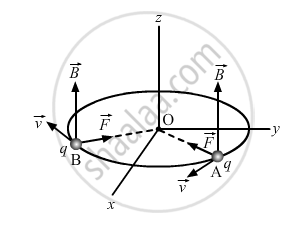Share

Deduce an Expression for the Frequency of Revolution of a Charged Particle in a Magnetic Field - CBSE (Science) Class 12 - Physics

ConceptMotion in Combined Electric and Magnetic Fields Cyclotron

Question

Deduce an expression for the frequency of revolution of a charged particle in a magnetic field and show that it is independent of velocity or energy of the particle.

Solution

When a charged particle with charge q moves inside a magnetic field vecBwith velocity v, it experiences a force, which is given by:

vecF=q(vecvxxvecB)

Here, vecv is perpendicular to vecB,vecFis the force on the charged particle which acts as the centripetal force and makes it move along a circular path.Let m be the mass of the charged particle and r be the radius of the circular path.

:.q(vecvxxvecB)=(mv^2)/r

v and B are at right angles:

:.qvB=(mv^2)/r

r=(mv)/(Bq)

Time period of circular motion of the charged particle can be calculated as shown below:

T=(2pir)/v

=(2pi)/v(mv)/(Bq)

T=(2pim)/(Bq)

∴ Angular frequency is

omega=(2pi)/T

 :.omega=(Bq)/m

Therefore, the frequency of the revolution of the charged particle is independent of the velocity or the energy of the particle.

Is there an error in this question or solution?

Video TutorialsVIEW ALL 

Solution Deduce an Expression for the Frequency of Revolution of a Charged Particle in a Magnetic Field Concept: Motion in Combined Electric and Magnetic Fields - Cyclotron.
S